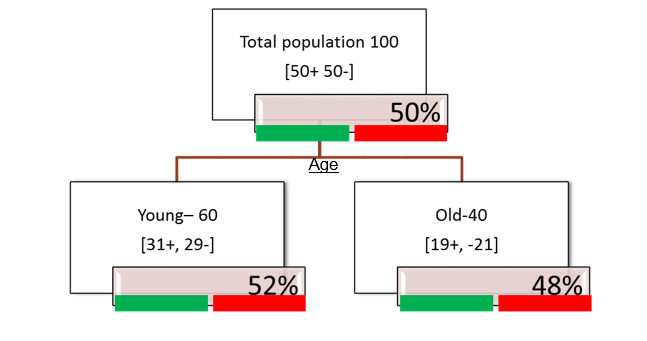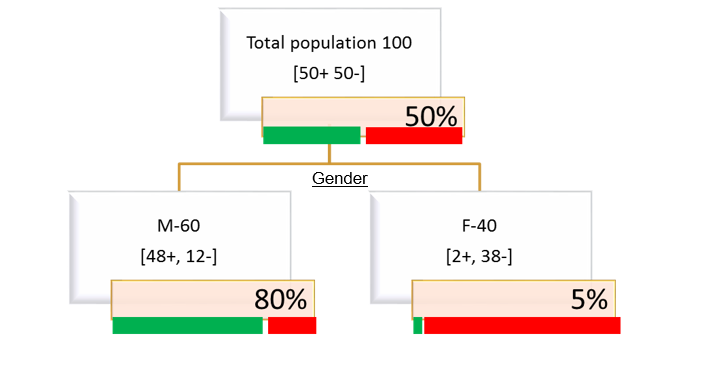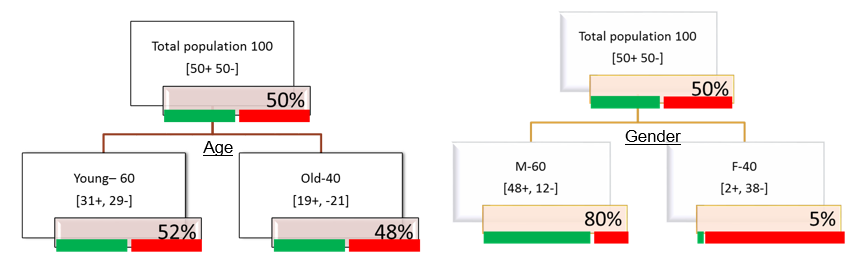• No products in the cart.

# 203.3.3 How Decision tree Splits works?

### The Splitting Criterion

In previous section, we studied about The Decision Tree Approach

• The best split is
• The split that does the best job of separating the data into groups
• Where a single class(either 0 or 1) predominates in each group

#### Example Sales Segmentation Based on Age#### Example Sales Segmentation Based on Gender## Impurity (Diversity) Measures

• We are looking for a impurity or diversity measure that will give high score for this Age variable(high impurity while segmenting), Low score for Gender variable(Low impurity while segmenting)• Entropy: Characterizes the impurity/diversity of segment
• Measure of uncertainty/Impurity
• Entropy measures the information amount in a message
• S is a segment of training examples, p+ is the proportion of positive examples, p- is the proportion of negative examples
• Entropy(S) = \(-p_+ log_2p_+ – p_- log_2 p_-\)
• Where \(p_+\) is the probabailty of positive class and \(p_-\) is the probabailty of negative class
• Entropy is highest when the split has p of 0.5.
• Entropy is least when the split is pure .ie p of 1

### Entropy is highest when the split has p of 0.5

• Entropy(S) = \(-p_+ log_2p_+ – p_- log_2 p_-\)
• Entropy is highest when the split has p of 0.5
• 50-50 class ratio in a segment is really impure, hence entropy is high
• Entropy(S) = \(-p_+ log_2p_+ – p_- log_2 p_-\)
• Entropy(S) = \(-0.5*log_2(0.5) – 0.5*log_2(0.5)\)
• Entropy(S) = 1### Entropy is least when the split is pure .ie p of 1

• Entropy(S) = \(-p_+ log_2p_+ – p_- log_2 p_-\)
• Entropy is least when the split is pure ie p of 1
• 100-0 class ratio in a segment is really pure, hence entropy is low
• Entropy(S) = \(-p_+ log_2p_+ – p_- log_2 p_-\)
• Entropy(S) = \(-1*log_2(1) – 0*log_2(0)\)
• Entropy(S) = 0### The less the entropy, the better the split

• The less the entropy, the better the split
• Entropy is formulated in such a way that, its value will be high for impure segments

The next post is about How to Calculate Entropy for Decision Tree Split.

20th June 2017
0 responses on "203.3.3 How Decision tree Splits works?"
© 2020. All Rights Reserved.